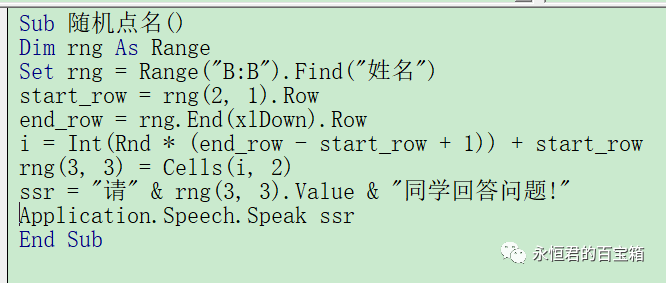﻿ Excel VBA 实例（25） – 班级随机点名并播报 - 永恒君的百宝箱

# Excel VBA 实例（25） – 班级随机点名并播报

517℃

``````=INDEX(A:A,RANDBETWEEN(2,15))
A:A为姓名的范围，RANDBETWEEN()随机抽取的行号
````````````Sub 随机点名()
Dim rng As Range
Set rng = Range("B:B").Find("姓名")
start_row = rng(2, 1).Row
end_row = rng.End(xlDown).Row
i = Int(Rnd * (end_row - start_row + 1)) + start_row
rng(3, 3) = Cells(i, 2)
ssr = "请" & rng(3, 3).Value & "同学回答问题!"
Application.Speech.Speak ssr
End Sub
``````

anyShare分享到：